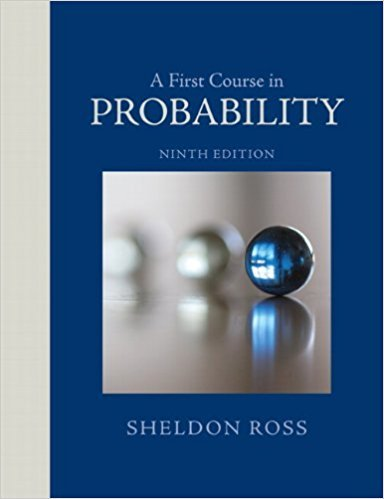×

×

# Solutions for Chapter 1: A First Course in Probability 9th Edition## Full solutions for A First Course in Probability | 9th Edition

ISBN: 9780321794772Solutions for Chapter 1

Solutions for Chapter 1
4 5 0 277 Reviews
24
4
##### ISBN: 9780321794772

Chapter 1 includes 77 full step-by-step solutions. This textbook survival guide was created for the textbook: A First Course in Probability , edition: 9. A First Course in Probability was written by and is associated to the ISBN: 9780321794772. Since 77 problems in chapter 1 have been answered, more than 63056 students have viewed full step-by-step solutions from this chapter. This expansive textbook survival guide covers the following chapters and their solutions.

Key Statistics Terms and definitions covered in this textbook

A formula used to determine the probability of the union of two (or more) events from the probabilities of the events and their intersection(s).

• Alternative hypothesis

In statistical hypothesis testing, this is a hypothesis other than the one that is being tested. The alternative hypothesis contains feasible conditions, whereas the null hypothesis speciies conditions that are under test

• Bernoulli trials

Sequences of independent trials with only two outcomes, generally called “success” and “failure,” in which the probability of success remains constant.

• Bias

An effect that systematically distorts a statistical result or estimate, preventing it from representing the true quantity of interest.

• Chi-square test

Any test of signiicance based on the chi-square distribution. The most common chi-square tests are (1) testing hypotheses about the variance or standard deviation of a normal distribution and (2) testing goodness of it of a theoretical distribution to sample data

• Coeficient of determination

See R 2 .

• Conditional probability

The probability of an event given that the random experiment produces an outcome in another event.

• Correction factor

A term used for the quantity ( / )( ) 1 1 2 n xi i n ? = that is subtracted from xi i n 2 ? =1 to give the corrected sum of squares deined as (/ ) ( ) 1 1 2 n xx i x i n ? = i ? . The correction factor can also be written as nx 2 .

• Defect concentration diagram

A quality tool that graphically shows the location of defects on a part or in a process.

• Defects-per-unit control chart

See U chart

• Discrete uniform random variable

A discrete random variable with a inite range and constant probability mass function.

• Eficiency

A concept in parameter estimation that uses the variances of different estimators; essentially, an estimator is more eficient than another estimator if it has smaller variance. When estimators are biased, the concept requires modiication.

• Empirical model

A model to relate a response to one or more regressors or factors that is developed from data obtained from the system.

• Factorial experiment

A type of experimental design in which every level of one factor is tested in combination with every level of another factor. In general, in a factorial experiment, all possible combinations of factor levels are tested.

• False alarm

A signal from a control chart when no assignable causes are present

• First-order model

A model that contains only irstorder terms. For example, the irst-order response surface model in two variables is y xx = + ?? ? ? 0 11 2 2 + + . A irst-order model is also called a main effects model

• Fractional factorial experiment

A type of factorial experiment in which not all possible treatment combinations are run. This is usually done to reduce the size of an experiment with several factors.

• Generating function

A function that is used to determine properties of the probability distribution of a random variable. See Moment-generating function

• Geometric random variable

A discrete random variable that is the number of Bernoulli trials until a success occurs.

• Hat matrix.

In multiple regression, the matrix H XXX X = ( ) ? ? -1 . This a projection matrix that maps the vector of observed response values into a vector of itted values by yˆ = = X X X X y Hy ( ) ? ? ?1 .